# Precise Inspection of Geometric Parameters for Polyvinyl Chloride Pipe Section Based on Computer Vision

Precise Inspection of Geometric Parameters for Polyvinyl Chloride Pipe Section Based on Computer Vision

Qilin Bi Minling Lai* Huiling Tang Yanyao Guo Jinyuan Li Xinhong Zeng Zhijun Liu

School of Navigation, Guangzhou Maritime University, Guangzhou 510725, China

School of Physics and Optoelectronic Engineering, Guangdong University of Technology, Guangzhou 510006, China

Zhejiang Ocean University, Zhoushan 316022, China

Corresponding Author Email:
2112115045@mail2.gdut.edu.cn
Page:
1647-1655
|
DOI:
https://doi.org/10.18280/ts.380608
17 August 2021
|
Accepted:
2 November 2021
|
Published:
31 December 2021
| Citation

OPEN ACCESS

Abstract:

The precise inspection of geometric parameters is crucial for quality control in the context of Industry 4.0. The current technique of precise inspection depends on the operation of professional personnel, and the measuring accuracy is restricted by the proficiency of operators. To solve the defects, this paper proposes a precise inspection framework for the geometric parameters of polyvinyl chloride (PVC) pipe section (G-PVC), using low-cost visual sensors and high-precision computer vision algorithms. Firstly, a robust imaging system was built to acquire images of a PVC pipe section under irregular illumination changes. Next, an engineering semantic model was established to calculate G-PVC like inner diameter, outer diameter, wall thickness, and roundness. After that, a region-of-interest (ROI) extraction algorithm was combined with an improved edge operator to obtain the coordinates of measured points on PVC end-face image in a stable and precise manner. Finally, our framework was proved highly precise and robust through experiments.

Keywords:

polyvinyl chloride (PVC) pipe, geometric parameters, visual inspection, region of interest (ROI), edge operator

1. Introduction

Polyvinyl chloride (PVC) pipes are widely applied in water supply, underground drainage, farmland irrigation, as well as mine and lab ventilation [1, 2], for their ease of construction, low price, and long service life. More than 60% of the global plastic pipe market is occupied by PVC pipes [3, 4].

The defects with the geometric parameters of PVC pipe section (G-PVC) affect the aesthetic experience, mechanical properties, and service life. Many factors could contribute to G-PVC: (1) The PVC pipe section deforms under the irregular changes of traction and extrusion speeds; (2) The variation of pipe thickness caused by impurities in the raw material [5, 6]; (3) The pipe section flattens due to the change of extrusion pressure.

Machine vision offers a non-contact, low-cost, and highly flexible technique to automatically inspect the quality of G-PVC. To reduce scrap rate and improve product quality, it is of great significance to realize precise inspection of G-PVC based on machine vision. By contrast, contact methods, which use vernier calipers, plug gauges, and sleeve rulers, might squeeze and deform the PVC end-face, and end up with a low inspection accuracy.

With the continuous update of detection technology, many attempts have been made to integrate new sensing technologies into G-PVC inspection. For example, SIKORA  developed a detection technology based on millimeter waves: On the outer circumference of the target pipe, multiple static/rotating transceivers are deployed to send and receive modulated millimeter waves at a high frequency; Then, the static system measures the wall thickness, inner diameter, and outer diameter selectively. However, this technology is cost sensitive, and only applicable to pipe end-face larger than 120mm in diameter. Zhang et al.  performed non-contact online measurement of PVC pipes with a laser diameter gauge. Their measuring approach raises a high requirement on laser beams. Gao et al.  created an ultrasonic online thickness measurement system for PVC pipe extruders, but the detection accuracy was hindered by the poor design of ultrasonic probe.

The detection of geometric parameters is a common task in pipe manufacturing . There are mature strategies for geometric parameter detection, including image difference methods [11, 12], connected domain detection [13, 14], image segmentation [15, 16], and edge detection [17, 18]. However, there is no approach that suits all scenarios. Different computer vision systems and algorithms should be selected for different tasks.

Computer vision has already been leveraged for inspection, which is essential to the control of manufacturing quality [19, 20]. Hou et al.  designed a machine vision system to inspect the size of Xuesaitong Dropping Pills (XDPs), and thus evaluated the appearance quality of XDPs rapidly and accurately. Li et al.  analyzed the gray feature of fusion hole images (FHIs), and designed an interference rejection algorithm for weld pool images; the geometric size was measured in line with the edge feature of the target FHI. Song and Wu  hailed geometric template matching as an ideal method to obtain location geometries of printed board surface, and pointed out the defects of the approach: it is difficult to generalize, and not involved in the improvement of geometric parameter inspection and classification. The above research has been improved in the following aspects:

(1) Hardware improvements

To improve the accuracy of cylindrical part inspection, Mills and Pitchford  obtained cylindrical part images with high-resolution line-scan cameras. Focusing on the straightness inspection of seamless steel pipes, Lu et al.  projected laser onto the steel pipe surface, obtained the geometric center of each arc, and fitted it into a straight line. Chen  employed chain code technology to speed up the visual inspection of circularity of non-continuous arcs. Wang et al.  designed an integrated mobile robotic system for measuring large-scale components with complex curved surfaces. The system, consisting of a mobile manipulator, a fringe projection scanner, and a stereo vision system, enables accurate noncontact three-dimensional (3D) measurements of large-scale complex components. Jeong et al.  introduced a conditionally paired generative network to generate synthetic images of scarce defects under four different lighting conditions, and significantly improved the accuracy of detecting such defects.

(2) Software improvements

For automatic inspection of circuit boards, Lahajnar et al.  utilized sub-pixel edge detection technology and semi-automatic correction system to distinguish the physical features of the circuit board in the machine vision system. Li et al.  designed a real-time inspection network for electronic components based on the matching between effective receptive field size and anchor size in you only look once (YOLO)v3. The network recognizes the location geometries on the printed circuit board (PCB) based on computer vision, despite the small size and similar appearance of electronic components on the PCB. The above attempts indeed improved the detection accuracy to a certain extent, but did not achieve a high efficiency. To improve real-time performance, Tech and Mital  invented an automatic vision system based on parallel algorithms, and demonstrated the high accuracy and fast speed of the system: it only took 1.5s for the system to detect each component.

In this paper, a hardware imaging system is synthetized with several efficient computer vision algorithm into a precise inspection framework for G-PVC. The main contributions are as follows:

(1) The proposed G-PVC precision inspection system can work flexibly on workpieces of different sizes, and facilitate the quality control of PVC pipes.

(2) Relying on image features, our novel mathematical model adapts well to the flexible process of G-PVC detection, making the detection results more reliable and accurate.

(3) Efficient image segmentation and region-of-interest (ROI) detection methods were improved to recognize the detected feature points in the target image accurately and efficiently.

Consequently, a G-PVC inspection prototype is built for industrial applications. Experimental results show that our method can obtain G-PVC images stably under irregular changes of natural light; the proposed G-PVC mathematical model can output precise expressions for different pipe diameters and detection accuracies; ROI and its parameter optimization algorithm can ensure the stable and precise extraction of feature points, while reducing the computing load, and thereby improve G-PVC detection efficiency; the improved Canny algorithm can stably pinpoint PVC contours in complex scenarios, such as background and burrs; the sum of squares for error (SSE) of our method fell within +0.013 in the G-PVC detection, the R-squared (RS) approached 0.995, the accuracy was increased by 80%, and the time consumption was reduced by 90% for the elimination of manual intervention.

The remainder of this paper is organized as follows: Section 2 briefly introduces the workflow of our computer vision-based G-PVC inspection system; Section 3 establishes the relationship between G-PVC and coordinates of feature points; Section 4 details ROI recognition, ROI parameters optimization, and edge detection algorithms for inner and outer circles; Section 5 presents experimental results and discussions; Section 6 summarizes the research findings.

2. G-PVC Inspection System

Figure 1 shows the details of our G-PVC inspection system, which mainly contains such subsystems as optical imaging, information processing, mechanism actuator, and motion control. Among them, the optical imaging subsystem is composed of a charge-coupled device (CCD) camera, an auxiliary light source, and an imaging posture adjustment mechanism. Although backlight-based illumination can result in obvious features, it is difficult to assist the imaging of PVC pipe end-face with backlight, because the pipe might be several meters and even tens of meters in length. Hence, the coaxial light source was selected to enhance the auxiliary imaging system. The G-PVC inspection system works under the following principle:

Firstly, the PVC pipe is transported by the feeding device to the preset detection position. Once the object is detected by the position sensor, a trigger signal is sent to the CCD camera and the auxiliary light source to generate a PVC end-face image. Next, dynamic ROI and improved Canny operator are combined to efficiently pinpoint the coordinates of the measuring point in the image coordinate system. Finally, G-PVC is inspected precisely according to the coordinates of measuring points, the calibration parameters of the camera, and the mapping between G-PVC and the coordinates of measuring points.

## 1.jpg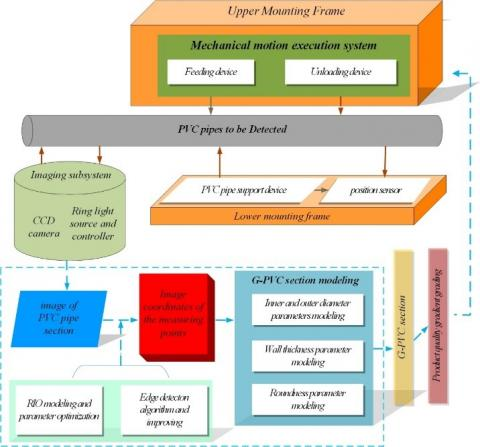Figure 1. Details on G-PVC inspection system

3. G-PVC Modeling

3.1 Modeling of internal and external diameters

During quality control, G-PVC affects the mechanical property and quality of the PVC end-face. The forming of PVC pipes is controlled by key parameters like internal and external diameters (Figure 2).

According to ISO3126:2005, the internal and external diameters of the PVC end-face are denoted as d and D, respectively; the number of detection points as 2n; the number of measured diameters as n; the coordinates of the mean center points in internal and external diameters as (uD0, vD0) and (ud0, vd0), respectively.

As per the requirements on PVC quality control, n must be positively proportional to D. To obtain the first measured external diameter D1, a straight line is drawn across point (uD0+(m-2i) ε/m, vD0) parallel to axis v to intersect the circumscribed circle of the PVC end-face. In this way, a series of external diameters D1i can be generated. The first measured external diameter D1 can be established as:

${{D}_{1}}={{\left( {{D}_{1i}} \right)}_{\text{max}}},i=\text{0,1,2,3,}\cdot \cdot \cdot \text{,m}$             (1)

where, m is the division coefficient of offset ε, which is negatively correlated with the roundness of the circumscribed circle for the target end-face. The greater the m value, the better the calculation accuracy, and the longer the computing time.

As shown in Figure 2(b), the k-th external diameter DK (k=1,2,3,...,n) can be calculated by:

${{\theta }_{k}}=\frac{\pi \text{*}k}{n}$            (2)

Subsequently, a straight line passing point $\left(\mathrm{u}_{\mathrm{D} 0}+\frac{\mathrm{m}-2 \mathrm{i}}{\mathrm{m}} \varepsilon * \sin \theta_{\mathrm{k}}, \mathrm{v}_{\mathrm{D} 0}+\frac{\mathrm{m}-2 \mathrm{i}}{\mathrm{m}} \varepsilon^{*} \cos \theta_{\mathrm{k}}\right)$ is drawn with a slope of tanθk. The series of parallel lines drawn above intersect the circumscribed circle of the PVC pipe, producing a series of external diameters Dki, the k-th measured external diameter Dk can be given by:

${{D}_{k}}={{\left( {{D}_{ki}} \right)}_{max}},i=\text{0,1,2,3,}\cdot \cdot \cdot \text{,m}$           (3)

The k-th internal diameter dk of the inscribed circle of the PVC end-face can be obtained in a similar manner.

## 2.png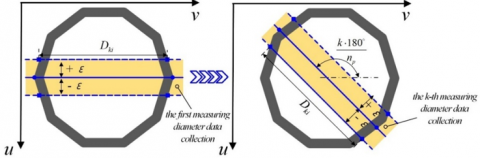Figure 2. Modeling of internal and external diameters

3.2 Modeling of thickness

Let Ak and ak be the intersections of the circumscribed circle and the straight line of the measured external diameter Dk; Bk and bk be the intersections of the inscribed circle and the straight line of the measured external diameter Dk; Aʹk and aʹk be the intersections of the circumscribed circle and the straight line of the measured internal diameter dk; Bʹk and bʹk be the intersections of the inscribed circle and the straight line of the measured internal diameter dk. All these intersections are shown in Figure 3. Then, the thickness δθk and δθk+π can be respectively expressed as:

\left\{ \begin{align} & {{\delta }_{{{\theta }_{k}}}}=\text{ }\!\![\!\!\text{ }({{A}_{k}}-{{a}_{k}}),(A_{k}^{'}-a_{k}^{'})\text{ }\!\!]\!\!\text{ min} \\ & {{\delta }_{{{\theta }_{k}}+\pi }}=\text{ }\!\![\!\!\text{ }({{B}_{k}}-{{b}_{k}}),(B_{k}^{'}-b_{k}^{'})\text{ }\!\!]\!\!\text{ min} \\\end{align} \right.          (4)

## 3.png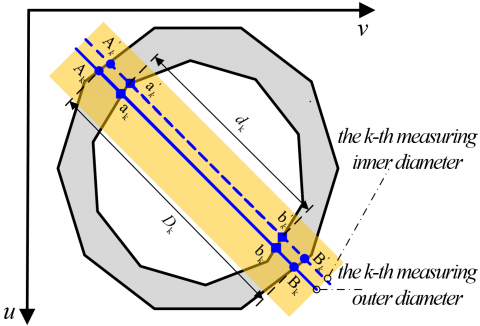Figure 3. Modeling of thickness

3.3 Modeling of roundness

Roundness is a key metric of the shape difference between an actual circle and the ideal circle. The tolerance zone of roundness refers to the area between two concentric circles, whose tolerance value equals radius difference. The roundness error can be evaluated by four types of methods, namely, minimum region method, least squares (LS) method, smallest circumscribed circle method, and largest inscribed circle method . The LS method stands out for its fast speed and stable performance. The accuracy of LS is restricted by the number of measured points: the more the points, the better the accuracy. In quality control, this paper adopts the LS method to calculate the roundness of PVC inner and outer circles, as well as the roundness errors. Let (uAk,vAk) and (uBk,vBk) be the coordinates of measuring points Ak and Bk at both ends of the k-th diameter to be measured in the circumscribed circle, respectively, with k=1,2,3,...,n. Then, the LS estimate of the ideal center (u0, v0) and the ideal radius R0 satisfies:

\left\{ \begin{align} & {{u}_{{{A}_{k}}}}-{{u}_{0}}{{}^{\text{2}}}+{{v}_{{{A}_{k}}}}-{{v}_{0}}{{}^{\text{2}}}\text{=}R_{0}^{2} \\ & {{u}_{{{B}_{k}}}}-{{u}_{0}}{{}^{\text{2}}}+{{v}_{{{B}_{k}}}}-{{v}_{0}}{{}^{\text{2}}}\text{=}R_{0}^{2} \\\end{align} \right.              (5)

Suppose αk1 =2(uAk+1-uAk), βk1=2(vAk+1-vAk), γk1=2(u2Ak+1+ v2Ak+1-u2Ak-u2Ak), αk2 =2(uBk+1-uBk), βk2=2(vBk+1-vBk), and γk2=2(u2Bk+1+ v2Bk+1-u2Bk -u2Bk). The center coordinates (u1,v1) can be obtained by the LS as:

\left\{ \begin{align} & {{u}_{1}}\text{=}\frac{1}{2}[\frac{\sum\limits_{i=1}^{m-1}{\beta _{k1}^{2}}\centerdot \sum\limits_{i=1}^{m-1}{{{\alpha }_{k1}}}{{\gamma }_{k1}}-\sum\limits_{i=1}^{m-1}{{{\beta }_{k1}}{{\gamma }_{k1}}}\centerdot \sum\limits_{i=1}^{m-1}{{{\alpha }_{k1}}}{{\beta }_{k1}}}{\sum\limits_{i=1}^{m-1}{\alpha _{k1}^{2}\centerdot \sum\limits_{i=1}^{m-1}{\beta _{k1}^{2}}\text{-}\sum\limits_{i=1}^{m-1}{{{\alpha }_{k1}}}{{\beta }_{k1}}{{}^{2}}}}+\frac{\sum\limits_{i=1}^{m-1}{\beta _{k2}^{2}}\centerdot \sum\limits_{i=1}^{m-1}{{{\alpha }_{k2}}}{{\gamma }_{k2}}-\sum\limits_{i=1}^{m-1}{{{\beta }_{k2}}{{\gamma }_{k2}}}\centerdot \sum\limits_{i=1}^{m-1}{{{\alpha }_{k2}}}{{\beta }_{k2}}}{\sum\limits_{i=1}^{m-1}{\alpha _{k2}^{2}\centerdot \sum\limits_{i=1}^{m-1}{\beta _{k2}^{2}}\text{-}\sum\limits_{i=1}^{m-1}{{{\alpha }_{k2}}}{{\beta }_{k2}}{{}^{2}}}}] \\ & {{v}_{1}}\text{=}\frac{1}{2}[\frac{\sum\limits_{i=1}^{m-1}{\alpha _{k1}^{2}}\centerdot \sum\limits_{i=1}^{m-1}{{{\beta }_{k1}}}{{\gamma }_{k1}}-\sum\limits_{i=1}^{m-1}{{{\alpha }_{k1}}{{\gamma }_{k1}}}\centerdot \sum\limits_{i=1}^{m-1}{{{\alpha }_{k1}}}{{\beta }_{k1}}}{\sum\limits_{i=1}^{m-1}{\alpha _{k1}^{2}\centerdot \sum\limits_{i=1}^{m-1}{\beta _{k1}^{2}}\text{-}\sum\limits_{i=1}^{m-1}{{{\alpha }_{k1}}}{{\beta }_{k1}}{{}^{2}}}}+\frac{\sum\limits_{i=1}^{m-1}{\alpha _{k2}^{2}}\centerdot \sum\limits_{i=1}^{m-1}{{{\beta }_{k2}}}{{\gamma }_{k2}}-\sum\limits_{i=1}^{m-1}{{{\alpha }_{k2}}{{\gamma }_{k2}}}\centerdot \sum\limits_{i=1}^{m-1}{{{\alpha }_{k2}}}{{\beta }_{k2}}}{\sum\limits_{i=1}^{m-1}{\alpha _{k2}^{2}\centerdot \sum\limits_{i=1}^{m-1}{\beta _{k2}^{2}}\text{-}\sum\limits_{i=1}^{m-1}{{{\alpha }_{k2}}}{{\beta }_{k2}}{{}^{2}}}}] \\ \end{align} \right.             (6)

The radius R1 can be obtained by LS as:

$R_{l}=\frac{1}{2 n}\left[\sum_{k=1}^{n} \sqrt{\left(u_{A_{k}}-u_{1}\right)^{2}+\left(v_{A_{k}}-v_{1}\right)^{2}}+\sqrt{\left(u_{B_{k}}-u_{1}\right)^{2}+\left(v_{B_{k}}-v_{1}\right)^{2}}\right]$        (7)

Then, the maximum radius Rmax and minimum radius Rmin of the circumscribed circle on the PVC end-face can be discriminated separately as:

\left\{ \begin{align} & {{R}_{\text{max}}}={{\text{ }\!\![\!\!\text{ }{{\left| \overrightarrow{{{A}_{k}}{{O}_{1}}} \right|}_{\text{max}}}\text{,}{{\left| \overrightarrow{{{B}_{k}}{{O}_{1}}} \right|}_{\max }}\text{ }\!\!]\!\!\text{ }}_{\text{max}}},k=\text{1,2,3,}\cdot \cdot \cdot \text{,n} \\ & {{R}_{\text{min}}}={{\text{ }\!\![\!\!\text{ }{{\left| \overrightarrow{{{A}_{k}}{{O}_{1}}} \right|}_{\text{min}}}\text{,}{{\left| \overrightarrow{{{B}_{k}}{{O}_{1}}} \right|}_{\min }}\text{ }\!\!]\!\!\text{ }}_{\text{min}}},k=\text{1,2,3,}\cdot \cdot \cdot \text{,n} \\ \end{align} \right.            (8)

where, Ak and Bk are the measured points on the PVC circumscribed circle; O1 is the center coordinate of the LS circle fitted by the measuring points on the circumscribed circle. Hence, the roundness of the circumscribed circle of the PVC end-face can be calculated by:

${{\varphi }_{R}}\text{=}\frac{{{R}_{\text{max}}}\text{-}\quad{{R}_{\text{min}}}}{{{R}_{1}}}$            (9)

The roundness of the inscribed circle of the PVC end-face can be calculated in a similar manner.

4. G-PVC Inspection Strategy

4.1 ROI localization and width determination

The results of G-PVC inspection hinges on the precise extraction of the intersection diameters and the inner and outer circles. This paper designs a feature point extraction algorithm based on dynamic ROI and edge operators (Figure 4). Specifically, a dynamic ROI of the size a (pixel) ×b (pixel) is created at the center point (uD0, vD0), where b=η*Dk is parallel to the straight line of outer diameter Dk. To reduce the computing load, the η value is controlled between 1.1 and 1.2, such that the ROI must intersect with the circumscribed circle.

The dynamic ROI is close to the k-th measured diameter of the circumscribed circle. A small ROI size helps to reduce the computing load and improve the computing efficiency. If the ROI size is small, however, it is possible that the ROI does not intersect with the circumscribed circle, or the intersection between them is unstable. For example, when a is 1 pixel, the ROI does not intersect with the circumscribed circle (Figure 4(a)); when a is 2 pixels, the intersection might be above or below the ROI (Figures 4(c) and (d)).

To ensure the uniqueness of the intersection, the value of a must be an odd number. As shown in Figure 4(e), when a is 3 pixels, the intersection remains stable, and reflects the end point of the measured diameter. In this case, the ROI expands with the growth of the size parameter a, and the end point of the measured diameter can be acquired with a high anti-interference ability and accuracy. Nevertheless, a=3 pixels bring a large computing load and a low computing efficiency. Through overall consideration of stability, efficiency and accuracy of feature point extraction, this paper sets a to 3 pixels.

## 4.jpgFigure 4. End point of the measured diameter

4.2 Edge operator

The traditional Canny operator is an excellent tool for contour recognition. When it comes to contour detection of PVC end-face, the blurry edges often lead to a high error rate of recognition, and an undesired positioning accuracy. To improve the recognition effect, this paper replaces the original 2×2 template with a 3×3 template, so that the central pixel gradient is completed by more pixels. Our edge detection algorithm is as follows:

(1) Let f(i, j) be the gray value of pixel (i, j) in a digital image; px and py be the gradients along horizontal and vertical directions, respectively; M (i, j) and a(i, j) be the magnitude and direction of the gradient at a point, respectively (Figure 5). The gradient of the gray value at point (i, j) can be obtained through first-order finite difference approximation:

\left\{ \begin{align} & {{p}_{x}}=\frac{\partial f}{\partial x}=\frac{\Delta f}{\Delta x} \\ & {{p}_{y}}=\frac{\partial f}{\partial y}=\frac{\Delta f}{\Delta y} \\ \end{align} \right.             (10)

## 5.jpgFigure 5. Gray value distribution

(2) In our improved Canny operator, the 3×3 convolution template can be expressed as:

$p_{x}=\left[\begin{array}{l}-1,-1,-1 \\ 0,0,0 \\ 1,1,1\end{array}\right], p_{\mathrm{y}}=\left[\begin{array}{l}-1,0,1 \\ -1,0,1 \\ -1,0,1\end{array}\right]$          (11)

(3) According to Figure 5, the gradients px and py at pixel (i, j) can be respectively expressed as:

$\left\{\begin{array}{l}p_{x}(i, j)=[(f(i+1, j-1)-f(i-1, j-1)] \\ +[f(i+1, j)-f(i-1, j)] \\ +[f(i+1, j+1)-f(i+1, j+1)] \\ p_{y}(i, j)=[f(i-1, j+1)-f(i-1, j-1)] \\ +[f(i, j+1]-f(i, j-1)] \\ +[f(i+1, j+1)-f(i+1, j-1)]\end{array}\right.$          (12)

(4) Through the reasoning above, the magnitude M(i,j) and direction ɑ(i, j) of the gradient satisfy:

\left\{ \begin{align} & M(i,j)=\sqrt{{{\text{ }\!\![\!\!\text{ }{{p}_{x}}(i,j)\text{ }\!\!]\!\!\text{ }}^{2}}+{{\text{ }\!\![\!\!\text{ }{{p}_{y}}(i,j)\text{ }\!\!]\!\!\text{ }}^{2}}} \\ & \partial (i,j)=\arctan \frac{{{p}_{y}}(i,j)}{{{p}_{x}}(i,j)} \\ \end{align} \right.              (13)

5. Experiments and Results Analysis

To verify its performance, the proposed G-PVC inspection method was compared with the approach of ultrasonic measurement  on our self-designed computer vision-based detection platform, which contains a camera (MER-050-200GM/C, 1,024 pixel×1,280 pixel), a visual lens (COMPUTAR, fD=6.5 mm), a customized white strip light, and a light controller (Advantech IPC, AiMC/3202). In addition, a software system was developed based on OpenCV  for visual inspection (Figure 6(a)). The inspection methods were implemented and evaluated on the same platform.

5.1 Experiments setup

Our experiments target the PVC pipe with four different diameters (Each of the two types of pipes has an inner diameter and an outer diameter. In total, there are four diameters), and test parameters like outer diameter, inner diameter, wall thickness, roundness, etc. (Figure 6(b)). Firstly, the internal and external parameters of the camera were calibrated by the method of AAA proposed by Bi et al. . Secondly, the parameters of the edge operator and ROI were adjusted to ensure the contrast of the edge features between internal and external circles, and obtain the intersection features stably and accurately. Thirdly, G-PVC was computed by formulas (3) (4) and (9), according to the internal and external parameters of the camera. Finally, the results of our approach were compared with those of mature detection methods.

Contact measurement has long been adopted in practice to measure the geometries of end faces. However, the measuring accuracy is often undermined by poor contact or the deformation induced by excessive contact force. To solve the problem, PVC pipe with four different diameters were selected for comparative experiments.

## 6a.png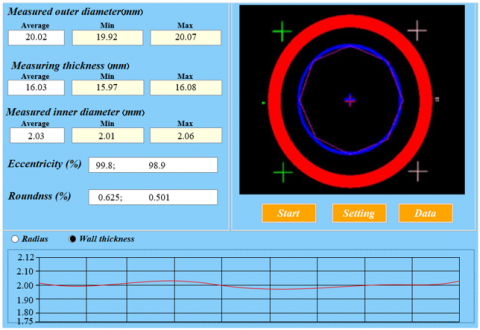(a) Software interface

## 6b.png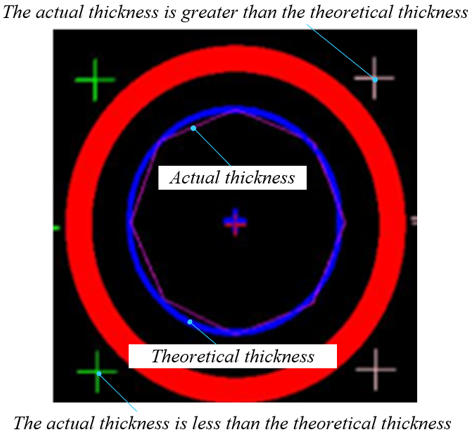(b) Location of measuring points and parameters

Figure 6. G-PVC detection process

5.2 Edge extraction performance

Figures 6 compares the edge extraction performance between the traditional Canny operator and our improved Canny operator. It can be observed that our method filtered out the edge artifacts caused by auxiliary light sources better than the traditional method. Our method also suppressed edge defects like burrs and shadows, laying a solid basis for accurate and stable defect inspection. On running time, the traditional Canny operator consumed an average of 0.0123s to edge an object contour, while our operator took only 0.0152s. Hence, the complexity of our contour detection strategy is affordable. Furthermore, with the increase of a value, the recognition results basically remained constant, while the computing time rose from 0.0081s to 0.0292 s.

5.3 G-PVC inspection performance

## 7.png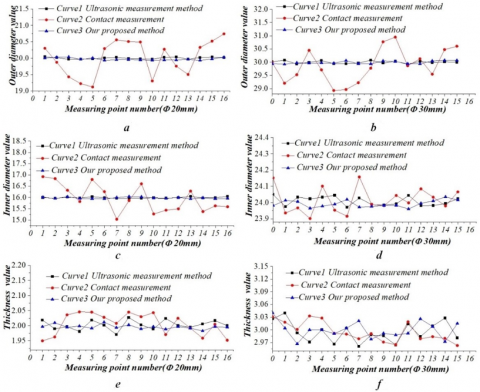Figure 7. G-PVC inspection by different methods

For rigid round PVC pipe sections, G-PVC can be measured by the mature ultrasonic technique. In this paper, two types of PVC pipes are selected for inspection: D0=20mm, δ0=2mm; D0=30mm, δ0=3mm. Experiments were carried out on these pipes to compare traditional methods with our method. The results of different methods are shown in Figure 7.

Ultrasonic method outputted similar results as our method, but the results of the traditional contact method deviated far from those of other methods. The main reason is that the PVC end-face suffers deformation or poor contact induced by the fluctuating contact force during the inspection. That is why the traditional contact method has a poor accuracy.

Besides, the deviation of the traditional vernier caliper method increased with the radius of the pipe. This is because the growing pipe diameter weakens the rigidity, which in turn magnifies deformation and measuring error.

Meanwhile, the increase of pipe diameter widened the relative measuring difference between our method and the ultrasonic method, indicating that the measuring accuracy of our method decreases with the growth in the geometric size of the pipe section. The measuring error of our method increases, mainly because a larger section means a broader field of view, and a poorer imaging quality. To improve the measuring accuracy, the camera resolution must be in line with the diameter of the target end face.

5.4 Overall performance

The performance of our method was evaluated by SSE, root mean square error (RMSE), and RS.

(1) The SSE was employed to evaluate the measuring error. The smaller the SSE, the better the detection accuracy. The SSE can be calculated by:

$SSE=\left[ \sum{{{\omega }_{i}}{{\text{(}{{x}_{i}}-{{x}_{i0}}\text{)}}^{2}}} \right]$               (14)

(2) The RMSE was adopted to estimate the square root of the ratio of the SSE between the measured value and the true value. The closer the RMSE is to 1, the greater the gap between the two values. The inverse is also true. The RMSE can be calculated by:

$RMSE=\sqrt{\frac{1}{k}\sum{{{\omega }_{i}}{{({{x}_{i}}-{{x}_{i0}})}^{2}}}}$             (15)

(3) The RS was chosen to depict the measuring accuracy. The closer RS is to 1, the more accurate the measurement. The RS can be calculated by:

${{R}_{s}}=1-\frac{\sum{{{\omega }_{i}}{{({{x}_{i}}-{{x}_{i0}})}^{2}}}}{\sum{{{\omega }_{i}}{{({{x}_{i}}-\overline{{{x}_{i}}})}^{2}}}}$             (16)

where, ωi is the weight coefficient; xi is the measured value; xi0 is the true value; $\overline{\mathrm{X}_{1}}$ is the mean.

The softness of PVC and the ultrasonic error make it difficult to detect the true size of PVC pipes with the existing methods. To verify the correctness of our model and algorithm, five types of steel pipes (D0=20mm, δ0=2mm, n=16; D0=40mm, δ0=3mm, n=24; D0=60mm, δ0=4mm, n=32; D0=80mm, δ0=6mm, n=48; D0=100mm, δ0=6mm, n=72) were selected as the test workpieces. The number n increases with the measuring diameter of the workpiece.

During the experiments, three-coordinate measuring machines (DAISY 564L precision: 0.0026mm) was utilized to measure each workpiece, and the measured value was approximated as the true value. The traditional ultrasonic measurement method and our method were separately introduced to measure the internal diameter, external diameter, wall thickness, and roundness of PVC. The measured results were evaluated by formulas (14)-(16). The evaluation parameters are given in Table 1. The following conclusions can be drawn:

(1) The SSE and RMSE fell within +0.013 and +0.026, respectively, a sign of a very small gap between the measured results of our method and the true value. The RS approximated 0.995, reflecting the high measuring accuracy of our method.

(2) With the growth of pipe diameter, SSE and RMSE increased, while the RS declined. That is, as the pipe diameter increased, the inspection became less accurate, and the error continued to increase. The main reason is that, the growing diameter of the PVC pipe reduces the pixel density in the image of PVC end-face. Thus, the positioning error of each measured point increases, and the G-PVC error of our method grows. To overcome the defect, the resolution of the camera needs to be enhanced, so as to keep the imaging pixel density of the PVC end-face unchanged. Then, the positioning error of the coordinates of each measurement point will no longer increase with pipe diameter. In this way, it is possible to measure large-diameter PVC accurately.

Table 1. Evaluation indices of different methods

 Parameters Metrics Ultrasonic method (Pipe diameter, mm) Our method (Pipe diameter, mm) 20 40 60 80 20 40 60 80 External diameter RMSE 2.863 2.881 2.891 2.932 2.982 3.354 3.563 4.023 RS 0.9962 0.9963 0.9961 0.9958 0.9952 0.9943 0.9936 0.9912 Internal diameter RMSE 3.151 3.171 3.163 3.203 4.214 4.561 4.782 4.821 RS 0.9952 0.9943 0.9958 0.9963 0.9922 0.9913 0.9899 0.9882 Thickness RMSE 3.863 3.877 3.872 3.965 5.199 5.311 5.629 5.873 RS 0.9912 0.9913 0.9942 0.9932 0.9898 0.9883 0.9872 0.9865 External roundness RMSE 3.231 3.256 3.342 3.381 3.420 3.612 3.842 3.981 RS 0.9942 0.9945 0.9951 0.9949 0.9931 0.9919 0.9902 0.9897 Internal roundness RMSE 3.318 3.498 3.392 3.419 3.685 3.802 3.925 3.996 RS 0.9921 0.9936 0.9948 0.9944 0.9881 0.9879 0.9862 0.9855
6. Conclusions

This paper proposes a flexible precision inspection system for G-PVC based on computer vision. The system can image G-PVC stably under irregular changes of natural light. Our mathematical model of the PVC pipe was developed against image features, providing a precise expression of G-PVC under different pipe diameters and different detection accuracies. Moreover, ROI and its parameter optimization algorithm were leveraged to eliminate interferences like background and glitches. By controlling the computing load, the authors realized stable and accurate extraction of feature points, and achieved efficient detection of G-PVC. Further, the improved Canny algorithm was adopted to extract the contours of PVC pipe end-face, such that the PVC contours could be recognized accurately and stably, in spite of background and burrs. Owing to the above innovations, our G-PVC inspection method achieve high performance and efficiency on steel pipes. Experimental results demonstrate the high flexibility of our method: the accuracy was increased by 80% and the running time was reduced by 90% in G-PVC inspection, thanks to the eliminating of manual intervention (the SSE was maintained within +0.013, and RS approximated 0.995). No parameter was measured incorrectly by our method throughout the inspection process. The future research will study the efficient and precise inspection of G-PVC with large diameters (greater than 200mm).

Acknowledgment

This paper is funded by Natural Science Foundation of Guangdong Province, China (Grant No.: 2021A1515010533); Science and Technology Department of Guangdong Province, China (Grant No.: 2017B010118004; 2020A050515003); Science and Technology Project of Guangzhou (Grant No.: 202002030463); Special Fund Project for Cultivating Science and Technology Innovation of University Students (Grant No.: pdjh2020b0442; pdjh2021a0378); Innovation and Entrepreneurship Education Project of Universities in Guangzhou (Grant No.: 2020HD002, 2019KTSCX129).

References

 Titow, M.V. (2012). PVC Technology. Springer Science & Business Media.

 Chao, K.P., Wang, P., Wang, B.H. (2016). Determining the permeability of organic solvents through PVC pipes using a direct SPME model. Polymer Testing, 56: 110-114. https://doi.org/10.1016/j.polymertesting.2016.09.028

 Onieva, C., Blázquez, A. (2018). PVC-O pipe innovations. Reinforced Plastics, 62(4): 216-218. https://doi.org/10.1016/j.repl.2017.06.088

 Pham, S.M., Thanh, T.D. (2017). A study on the welding line strength of composite parts with various venting systems in injection molding process. In Key Engineering Materials, 737: 70-76. https://doi.org/10.4028/www.scientific.net/KEM.737.70

 Kuliczkowska, E., Zwierzchowska, A. (2016). A qualitative analysis of early defects present in PVC-U sewers but not observed in rigid pipes. Tunnelling and Underground Space Technology, 56: 202-210. https://doi.org/10.1016/j.tust.2016.03.013

 Fellahi, S., Meddad, A., Fisa, B., Favis, B.D. (1995). Weldlines in injection-molded parts: A review. Advances in Polymer Technology: Journal of the Polymer Processing Institute, 14(3): 169-195. https://doi.org/10.1002/adv.1995.060140302

 Harold, S. (2017). Millimeter wave technology for precise measurement of diameter, ovality, wall thickness and sagging during tube making. Rubber World: The Technical Service Magazine for The Rubber Industry, 256(2): 26-28.

 Zhang, Z., Yang, Y., Hou, J., Gong, Y. (2020). Modeling and simulation on speed prediction of bypass pipeline inspection gauge in medium of water and crude oil. Measurement and Control, 53(9-10): 1851-1860. https://doi.org/10.1177/0020294020947123

 Gao, Y., Wang, X., Xie, L., Gong, W. (2020). Research on ultrasonic phased array inspection technology of small diameter tube based on Ultravision simulation technology. In Journal of Physics: Conference Series 1635(1): 012001. https://doi.org/10.1088/1742-6596/1635/1/012001

 Chen, X., Chen, X., Wei, J., Cai, X., Li, Y. (2021). Visual inspection method for inner wall of small hole based on internal reflection principle. In Journal of Physics: Conference Series, 1732(1): 012031. https://doi.org/10.1088/1742-6596/1732/1/012031

 Villamizar, J., Calderón, G., Carrillo, J., Bautista Rozo, L., Carrillo, J., Rueda, J., Castillo, J. (2021). Mimetic finite difference methods for restoration of fundus images for automatic detection of glaucoma suspects. Computer Methods in Biomechanics and Biomedical Engineering: Imaging & Visualization, 1-8. https://doi.org/10.1080/21681163.2021.1914733

 Zhang, L., Shi, J.F., Luo, X. (2007). An analysis of image-difference methods in moving target detection. Industrial Instrumentation and Automation Device, 35-38.

 Zhao, J., Yu, H., Gu, X., Wang, S. (2010). The edge detection of river model based on self-adaptive Canny Algorithm and connected domain segmentation. In 2010 8th World Congress on Intelligent Control and Automation, pp. 1333-1336. https://doi.org/10.1109/WCICA.2010.5554869

 Jain, A., Jain, J. (1978). Partial differential equations and finite difference methods in image processing--Part II: Image restoration. IEEE Transactions on Automatic Control, 23(5): 817-834. https://doi.org/10.1109/TAC.1978.1101881

 Shi, J., Wu, K., Yang, C., Deng, N. (2021). A method of steel bar image segmentation based on multi-attention U-Net. IEEE Access, 9: 13304-13313. https://doi.org/10.1109/ACCESS.2021.3052224

 Zhang, T.X., Peng, J.X., Li, Z.J. (1996). An adaptive image segmentation method with visual nonlinearity characteristics. IEEE Transactions on Systems, Man, and Cybernetics, Part B (Cybernetics), 26(4): 619-627. https://doi.org/10.1109/3477.517037

 Lu, S., Liu, Z., Chen, Y., Gao, Y. (2021). A novel subpixel edge detection method of pantograph slide in complicated surroundings. IEEE Transactions on Industrial Electronics, (99): 1-1. https://doi.org/10.1109/TIE.2021.3062276

 Qu, Y.D., Cui, C.S., Chen, S.B., Li, J.Q. (2005). A fast subpixel edge detection method using Sobel–Zernike moments operator. Image and Vision Computing, 23(1): 11-17. https://doi.org/10.1016/j.imavis.2004.07.003

 Jiang, Z., Wang, X., Huang, X., Li, H. (2021). Triangulate geometric constraint combined with visual-flow fusion network for accurate 6DoF pose estimation. Image and Vision Computing, 108: 104127. https://doi.org/10.1016/j.imavis.2021.104127

 Hua, G., Tan, X. (2017). Affine-invariant geometric constraints-based high accuracy simultaneous localization and mapping. Journal of Sensors, 2017: Article ID 1969351. https://doi.org/10.1155/2017/1969351

 Hou, Y., Cai, X., Miao, P., Li, S., Shu, C., Li, P., Li, Z. (2021). A feasibility research on the application of machine vision technology in appearance quality inspection of Xuesaitong dropping pills. Spectrochimica Acta Part A: Molecular and Biomolecular Spectroscopy, 258: 119787. https://doi.org/10.1016/j.saa.2021.119787

 Li, C., Gao, J., Cao, Y., Yan, X., Gui, X. (2019). Visual observation of fusion hole in thin plate TIG welding with a reserved gap. Journal of Manufacturing Processes, 45: 634-641. https://doi.org/10.1016/j.jmapro.2019.08.002

 Song, J., Wu, X. (2018). Research on visual detection and control system of three axis servo dispensing machine. In 2018 Eighth International Conference on Instrumentation & Measurement, Computer, Communication and Control (IMCCC), pp. 542-546. https://doi.org/10.1109/IMCCC.2018.00120

 Mills, R., Pitchford, N. (1991). Development of a line-scan camera for 2D high accuracy measurement. Machine Vis, 267-277.

 Lu, R.S., Li, Y.F., Yu, Q. (2001). On-line measurement of the straightness of seamless steel pipes using machine vision technique. Sensors and Actuators A: Physical, 94(1-2): 95-101. https://doi.org/10.1016/S0924-4247(01)00683-5

 Chen, M.C. (2002). Roundness measurements for discontinuous perimeters via machine visions. Computers in Industry, 47(2): 185-197. https://doi.org/10.1016/S0166-3615(01)00143-9

 Wang, J., Tao, B., Gong, Z., Yu, S., Yin, Z. (2021). A mobile robotic measurement system for large-scale complex components based on optical scanning and visual tracking. Robotics and Computer-Integrated Manufacturing, 67: 102010. https://doi.org/10.1016/j.rcim.2020.102010

 Jeong, E.Y., Kim, J., Jang, W.H., Lim, H.C., Noh, H., Choi, J.M. (2021). A more reliable defect detection and performance improvement method for panel inspection based on artificial intelligence. Journal of Information Display, 1-10. https://doi.org/10.1080/15980316.2021.1876174

 Lahajnar, F., Pernuš, F., Kovačič, S. (2002). Machine vision system for inspecting electric plates. Computers in Industry, 47(1): 113-122. https://doi.org/10.1016/S0166-3615(01)00134-8

 Li, J., Li, W., Chen, Y., Gu, J. (2021). A PCB electronic components detection network design based on effective receptive field size and anchor size matching. Computational Intelligence and Neuroscience, 2021: Article ID 6682710. https://doi.org/10.1155/2021/6682710

 Teoh, E.K., Mital, D.P. (1995). A transputer-based automated visual inspection system for electronic devices and PCBs. Optics and Lasers in Engineering, 22(3): 161-180. https://doi.org/10.1016/0143-8166(94)00044-B

 Liu, D., Zheng, P., Cao, M., Yin, H., Xu, Y., Zhang, L. (2021). A new method of roundness error evaluation based on twin support vector machines. Measurement Science and Technology, 32(7): 075008. https://doi.org/10.1088/1361-6501/abe5e5

 Luo, S.H., Luo, X.Y., Bi Q.L. (2019). Pipe detection equipment: CN, CN209639682U.

 Pulli, K., Baksheev, A., Kornyakov, K., Eruhimov, V. (2012). Real-time computer vision with OpenCV. Communications of the ACM, 55(6): 61-69. https://doi.org/10.1145/2184319.2184337

 Bi, Q., Liu, Z., Wang, M., Lai, M., Xiao, L., Yan, Y., Liu, X. (2017). An automatic camera calibration method based on checkerboard. Traitement du Signal, 34(3-4): 209. https://doi.org/10.3166/TS.34.209-226